0
213

Download Venn Diagram Questions for XAT PDF – XAT Venn Diagram questions PDF by Cracku. Practice XAT solved Venn Diagram Questions paper tests, and these are the practice question to have a firm grasp on the Venn Diagram topic in the XAT exam. Top 20 very Important Venn Diagram Questions for XAT based on asked questions in previous exam papers.  The XAT question papers contain actual questions asked with answers and solutions.

Question 1: Study the given Venn diagram carefully and answer the following question.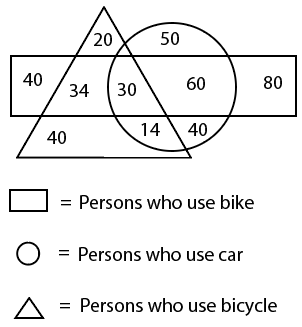How many persons use both a bicycle and a bike but not a car?

a) 30

b) 14

c) 34

d) 40

Solution:

From the Venn diagram,

Number of persons who use both bicycle and bike but not car = Intersection triangle and rectangle but not circle = 34

Hence, the correct answer is Option C

Question 2: Select the Venn diagram that best represents the relationship between the following classes.
Dirham, USD, Currency

a)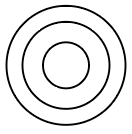b)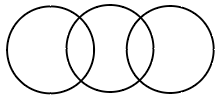c)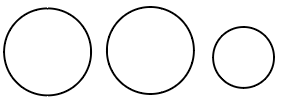d)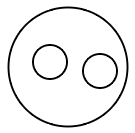Solution:

Dirham and USD are different currencies of different countries.

$\therefore\$The Venn diagram that best represents the relationship between the given classes is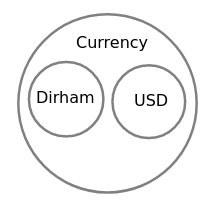Hence, the correct answer is Option D

Question 3: Select the Venn diagram that best represents the relationship between the following classes.
Infants, Males, Fathers

a)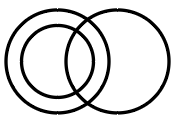b)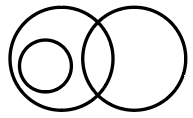c)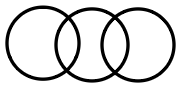d)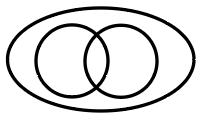Solution:

All Fathers are Males. Some Infants are Males. Infants cannot be Fathers.

$\therefore\$The Venn diagram that best represents the relationship between the given classes is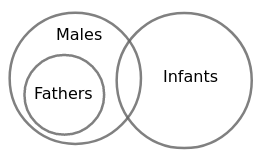Hence, the correct answer is Option B

Question 4: In a batch of 68 students, 23 students do not participate in any of the two games i.e. cricket and squash. 17 students participate in cricket only, 24 students participate in cricket and squash. How many students participate in squash only?

a) 28

b) 4

c) 21

d) 20

Solution:

Let the number of students who participate in squash only = a

The given information is represented in the Venn diagram as shown below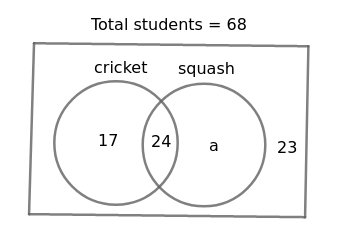From the Venn diagram,

17 + 24 + a + 23 = 68

$\Rightarrow$  64 + a = 68

$\Rightarrow$  a = 4

$\therefore\$Number of students who participate in squash only = 4

Hence, the correct answer is Option B

Question 5: Observe the following diagram and answer the question.
Find the number of students who play any two of the three sports.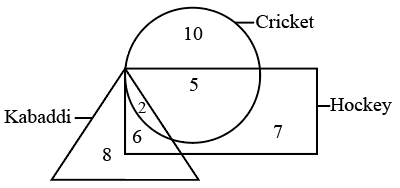a) 9

b) 15

c) 11

d) 13

Solution:

From the diagram,

Number of students who play any two of the three sports = Intersection of circle and rectangle + Intersection of triangle and rectangle = 5 + 6 = 11

Hence, the correct answer is Option C

Question 6: Select the Venn diagram that best illustrates the relationship between the following classes.
Fathers, Males, Brothers

a)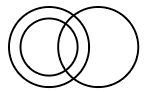b)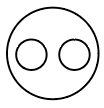c)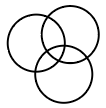d)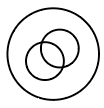Solution:

All fathers and brothers are males. Some fathers are brothers.

$\therefore\$The Venn diagram that best illustrates the relationship between the given classes is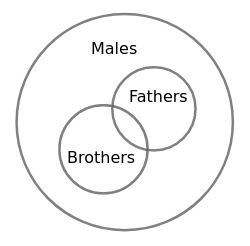Hence, the correct answer is Option D

Question 7: In the given Venn Diagram, ‘Circle’ represents ‘Players’, ‘Triangle’ represents ‘Graduates’ and ‘Rectangle’ represents ‘Managers’. Which of the numbers depicts the area which represents ‘Graduate Players who are not Managers’?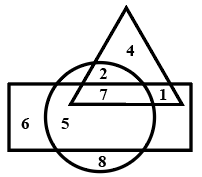a) 1

b) 5

c) 7

d) 2

Solution:

From the Venn diagram,

The number which represents ‘Graduate Players who are not Managers’ = Intersection of Triangle and Circle but not Rectangle = 2

Hence, the correct answer is Option D

Question 8: Select the Venn diagram that best represents the relationship between the following classes.
Physics, Science, Biology, Zoology

a)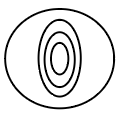b)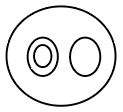c)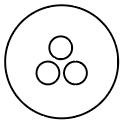d)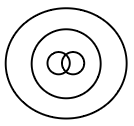Solution:

Physics, Biology comes under Science and Zoology comes under Biology.

$\therefore\$The Venn diagram that best represents the relationship between the given classes is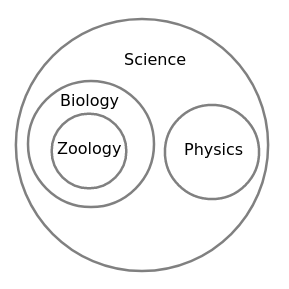Hence, the correct answer is Option B

Question 9: Select the Venn diagram that best represents the relationship between the following classes.
Tortoise, Mammal, Amphibian

a)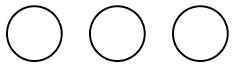b)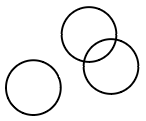c)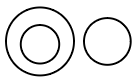d)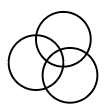Solution:

Mammals are the ones that feed their younger ones with milk.

Amphibians are the ones that can live both in land and water.

Tortoise is neither Mammal nor Amphibian.

$\therefore\$The Venn diagram that best represents the relationship between the given classes is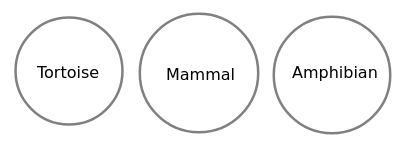Hence, the correct answer is Option A

Question 10: Study the following diagram and answer the given question.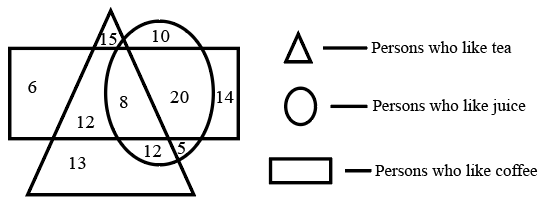How many people like both tea and coffee, but do NOT like juice?’

a) 22

b) 12

c) 8

d) 20

Solution:

From the diagram,

Number of people like both tea and coffee, but do not like juice = Intersection of triangle and rectangle but not circle = 12

Hence, the correct answer is Option B

Question 11: In the given Venn diagram, A likes milk, B likes bread and C likes meat. Who likes all the given eating materials?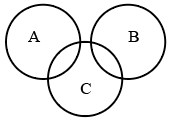a) Only B

b) Only C

c) Both B and C

d) Both A and B

Solution:

From the Venn diagram,

A has intersection with only C, so A likes milk and meat.

B has intersection with only C, so B likes bread and meat.

C has intersection with both A and B, so C likes meat, milk, bread.

$\therefore\$Only C likes all the given eating materials

Hence, the correct answer is Option B

Question 12: In the given Venn diagram, circle A represents books, circle B represents pens and circle C, represents pencils. Which number represents only books and pencils?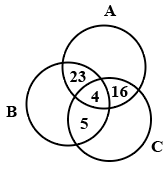a) 16

b) 23

c) 5

d) 20

Solution:

From the Venn Diagram,

Number which represents only books and pencils = Intersection of A and C but not B = 16

Hence, the correct answer is Option A

Question 13: In the given Venn diagram, A denotes mangoes, B denotes bananas, C denotes apples, and the numbers in each section represent the number of persons who like those fruits. How many persons like only bananas?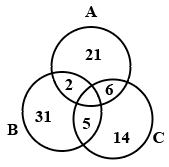a) 38

b) 7

c) 31

d) 33

Solution:

From the Venn diagram,

Persons who like only bananas = Only B without intersection of A and C = 31

Hence, the correct answer is Option C

Question 14: Study the following diagram.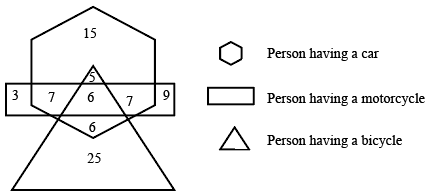How many persons have only motorcycle?

a) 32

b) 3

c) 9

d) 12

Solution:

From the given diagram,

Number of persons who have only a motorcycle = Number of persons in a rectangle with no intersection with hexagon and triangle = 3 + 9 = 12

Hence, the correct answer is Option D

Question 15: In the given Venn diagram, different animals are represented by different areas. ‘A’ denotes ‘dogs’, ‘B’ denotes ‘cats’, ‘C’ denotes ‘fishes’, ‘D’ denotes ‘mongooses’, ‘E’ denotes ‘rats’, and ‘F’ denotes ‘rabbits’. Which area represents the group of only dogs, fishes and mongooses?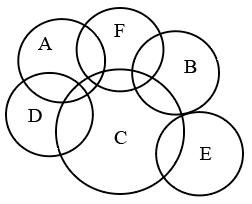a) D

b) F

c) C

d) A